# SciPy - Library of scientific algorithms for Python

J.R. Johansson (robert@riken.jp) http://dml.riken.jp/~rob/

The latest version of this IPython notebook lecture is available at http://github.com/jrjohansson/scientific-python-lectures.

The other notebooks in this lecture series are indexed at http://jrjohansson.github.com.

Welcome to pylab, a matplotlib-based Python environment [backend: module://IPython.zmq.pylab.backend_inline].


## Introduction

The SciPy framework builds on top of the low-level NumPy framework for multidimensional arrays, and provides a large number of higher-level scientific algorithms. Some of the topics that SciPy covers are:

Each of these submodules provides a number of functions and classes that can be used to solve problems in their respective topics.

In this lecture we will look at how to use some of these subpackages.

To access the SciPy package in a Python program, we start by importing everything from the scipy module.

If we only need to use part of the SciPy framework we can selectively include only those modules we are interested in. For example, to include the linear algebra package under the name la, we can do:

## Special functions

A large number of mathematical special functions are important for many computional physics problems. SciPy provides implementations of a very extensive set of special functions. For details, see the list of functions in the reference documention at http://docs.scipy.org/doc/scipy/reference/special.html#module-scipy.special.

To demonstrate the typical usage of special functions we will look in more detail at the Bessel functions:

J_0(0.000000) = 1.000000
Y_0(1.000000) = 0.088257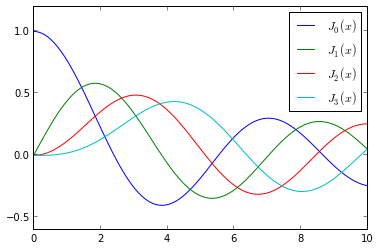array([  2.40482556,   5.52007811,   8.65372791,  11.79153444])


## Integration

Numerical evaluation of a function of the type

$\displaystyle \int_a^b f(x) dx$

is called numerical quadrature, or simply quadature. SciPy provides a series of functions for different kind of quadrature, for example the quad, dblquad and tplquad for single, double and triple integrals, respectively.

The quad function takes a large number of optional arguments, which can be used to fine-tune the behaviour of the function (try help(quad) for details).

The basic usage is as follows:

integral value = 0.5 , absolute error = 5.55111512313e-15


If we need to pass extra arguments to integrand function we can use the args keyword argument:

0.736675137081 9.38925687719e-13


For simple functions we can use a lambda function (name-less function) instead of explicitly defining a function for the integrand:

numerical  = 1.77245385091 1.42026367809e-08
analytical = 1.77245385091


As show in the example above, we can also use ‘Inf’ or ‘-Inf’ as integral limits.

Higher-dimensional integration works in the same way:

0.785398163397 1.63822994214e-13


Note how we had to pass lambda functions for the limits for the y integration, since these in general can be functions of x.

## Ordinary differential equations (ODEs)

SciPy provides two different ways to solve ODEs: An API based on the function odeint, and object-oriented API based on the class ode. Usually odeint is easier to get started with, but the ode class offers some finer level of control.

Here we will use the odeint functions. For more information about the class ode, try help(ode). It does pretty much the same thing as odeint, but in an object-oriented fashion.

To use odeint, first import it from the scipy.integrate module

A system of ODEs are usually formulated on standard form before it is attacked numerically. The standard form is:

$y’ = f(y, t)$

where

$y = [y_1(t), y_2(t), …, y_n(t)]$

and $f$ is some function that gives the derivatives of the function $y_i(t)$. To solve an ODE we need to know the function $f$ and an initial condition, $y(0)$.

Note that higher-order ODEs can always be written in this form by introducing new variables for the intermediate derivatives.

Once we have defined the Python function f and array y_0 (that is $f$ and $y(0)$ in the mathematical formulation), we can use the odeint function as:

y_t = odeint(f, y_0, t)


where t is and array with time-coordinates for which to solve the ODE problem. y_t is an array with one row for each point in time in t, where each column corresponds to a solution y_i(t) at that point in time.

We will see how we can implement f and y_0 in Python code in the examples below.

#### Example: double pendulum

Let’s consider a physical example: The double compound pendulum, described in some detail here: http://en.wikipedia.org/wiki/Double_pendulumThe equations of motion of the pendulum are given on the wiki page:

${\dot \theta_1} = \frac{6}{m\ell^2} \frac{ 2 p_{\theta_1} - 3 \cos(\theta_1-\theta_2) p_{\theta_2}}{16 - 9 \cos^2(\theta_1-\theta_2)}$

${\dot \theta_2} = \frac{6}{m\ell^2} \frac{ 8 p_{\theta_2} - 3 \cos(\theta_1-\theta_2) p_{\theta_1}}{16 - 9 \cos^2(\theta_1-\theta_2)}.$

${\dot p_{\theta_1}} = -\frac{1}{2} m \ell^2 \left [ {\dot \theta_1} {\dot \theta_2} \sin (\theta_1-\theta_2) + 3 \frac{g}{\ell} \sin \theta_1 \right ]$

${\dot p_{\theta_2}} = -\frac{1}{2} m \ell^2 \left [ -{\dot \theta_1} {\dot \theta_2} \sin (\theta_1-\theta_2) + \frac{g}{\ell} \sin \theta_2 \right]$

To make the Python code simpler to follow, let’s introduce new variable names and the vector notation: $x = [\theta_1, \theta_2, p_{\theta_1}, p_{\theta_2}]$

${\dot x_1} = \frac{6}{m\ell^2} \frac{ 2 x_3 - 3 \cos(x_1-x_2) x_4}{16 - 9 \cos^2(x_1-x_2)}$

${\dot x_2} = \frac{6}{m\ell^2} \frac{ 8 x_4 - 3 \cos(x_1-x_2) x_3}{16 - 9 \cos^2(x_1-x_2)}$

${\dot x_3} = -\frac{1}{2} m \ell^2 \left [ {\dot x_1} {\dot x_2} \sin (x_1-x_2) + 3 \frac{g}{\ell} \sin x_1 \right ]$

${\dot x_4} = -\frac{1}{2} m \ell^2 \left [ -{\dot x_1} {\dot x_2} \sin (x_1-x_2) + \frac{g}{\ell} \sin x_2 \right]$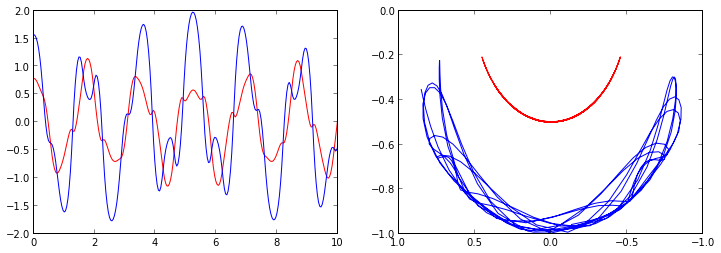Simple annimation of the pendulum motion. We will see how to make better animation in Lecture 4.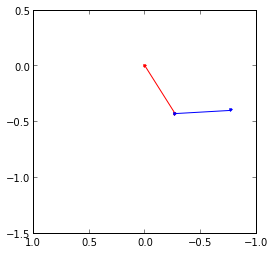#### Example: Damped harmonic oscillator

ODE problems are important in computational physics, so we will look at one more example: the damped harmonic oscillation. This problem is well described on the wiki page: http://en.wikipedia.org/wiki/Damping

The equation of motion for the damped oscillator is:

$\displaystyle \frac{\mathrm{d}^2x}{\mathrm{d}t^2} + 2\zeta\omega_0\frac{\mathrm{d}x}{\mathrm{d}t} + \omega^2_0 x = 0$

where $x$ is the position of the oscillator, $\omega_0$ is the frequency, and $\zeta$ is the damping ratio. To write this second-order ODE on standard form we introduce $p = \frac{\mathrm{d}x}{\mathrm{d}t}$:

$\displaystyle \frac{\mathrm{d}p}{\mathrm{d}t} = - 2\zeta\omega_0 p - \omega^2_0 x$

$\displaystyle \frac{\mathrm{d}x}{\mathrm{d}t} = p$

In the implementation of this example we will add extra arguments to the RHS function for the ODE, rather than using global variables as we did in the previous example. As a consequence of the extra arguments to the RHS, we need to pass an keyword argument args to the odeint function: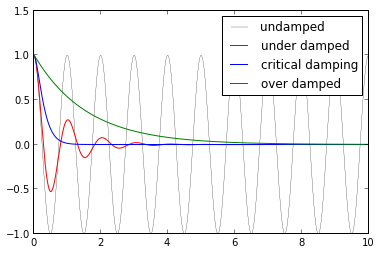## Fourier transform

Fourier transforms are one of the universal tools in computational physics, which appear over and over again in different contexts. SciPy provides functions for accessing the classic FFTPACK library from NetLib, which is an efficient and well tested FFT library written in FORTRAN. The SciPy API has a few additional convenience functions, but overall the API is closely related to the original FORTRAN library.

To use the fftpack module in a python program, include it using:

To demonstrate how to do a fast Fourier transform with SciPy, let’s look at the FFT of the solution to the damped oscillator from the previous section: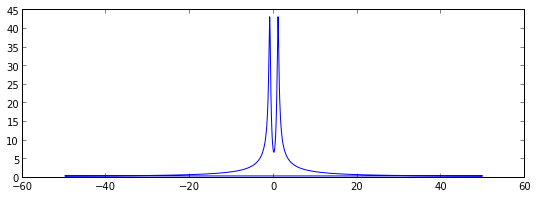Since the signal is real, the spectrum is symmetric. We therefore only need to plot the part that corresponds to the postive frequencies. To extract that part of the w and F we can use some of the indexing tricks for NumPy arrays that we saw in Lecture 2: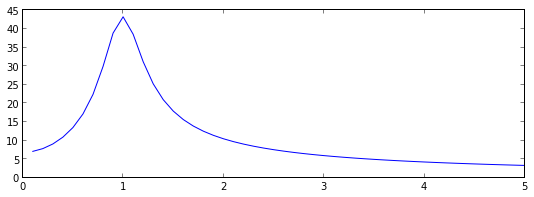As expected, we now see a peak in the spectrum that is centered around 1, which is the frequency we used in the damped oscillator example.

## Linear algebra

The linear algebra module contains a lot of matrix related functions, including linear equation solving, eigenvalue solvers, matrix functions (for example matrix-exponentiation), a number of different decompositions (SVD, LU, cholesky), etc.

Detailed documetation is available at: http://docs.scipy.org/doc/scipy/reference/linalg.html

Here we will look at how to use some of these functions:

### Linear equation systems

Linear equation systems on the matrix form

$A x = b$

where $A$ is a matrix and $x,b$ are vectors can be solved like:

array([-0.33333333,  0.66666667,  0.        ])

array([ -1.11022302e-16,   0.00000000e+00,   0.00000000e+00])


We can also do the same with

$A X = B$

where $A, B, X$ are matrices:

array([[ 2.28587973,  5.88845235,  1.6750663 ],
[-4.88205838, -5.26531274, -1.37990347],
[ 1.75135926, -2.05969998, -0.09859636]])

6.2803698347351007e-16


### Eigenvalues and eigenvectors

The eigenvalue problem for a matrix $A$:

$\displaystyle A v_n = \lambda_n v_n$

where $v_n$ is the $n$th eigenvector and $\lambda_n$ is the $n$th eigenvalue.

To calculate eigenvalues of a matrix, use the eigvals and for calculating both eigenvalues and eigenvectors, use the function eig:

array([ 1.06633891+0.j        , -0.12420467+0.10106325j,
-0.12420467-0.10106325j])

array([ 1.06633891+0.j        , -0.12420467+0.10106325j,
-0.12420467-0.10106325j])

array([[ 0.89677688+0.j        , -0.30219843-0.30724366j,
-0.30219843+0.30724366j],
[ 0.35446145+0.j        ,  0.79483507+0.j        ,  0.79483507+0.j        ],
[ 0.26485526+0.j        , -0.20767208+0.37334563j,
-0.20767208-0.37334563j]])


The eigenvectors corresponding to the $n$th eigenvalue (stored in evals[n]) is the $n$th column in evecs, i.e., evecs[:,n]. To verify this, let’s try mutiplying eigenvectors with the matrix and compare to the product of the eigenvector and the eigenvalue:

1.3964254612015911e-16


There are also more specialized eigensolvers, like the eigh for Hermitian matrices.

### Matrix operations

array([[-1.38585633,  1.36837431,  6.03633364],
[ 3.80855289, -4.76960426, -5.2571037 ],
[ 0.0689213 ,  2.4652602 , -2.5948838 ]])

0.027341548212627968

(1.1657807164173386, 1.7872032588446576)


### Sparse matrices

Sparse matrices are often useful in numerical simulations dealing with large systems, if the problem can be described in matrix form where the matrices or vectors mostly contains zeros. Scipy has a good support for sparse matrices, with basic linear algebra operations (such as equation solving, eigenvalue calculations, etc).

There are many possible strategies for storing sparse matrices in an efficient way. Some of the most common are the so-called coordinate form (COO), list of list (LIL) form, and compressed-sparse column CSC (and row, CSR). Each format has some advantanges and disadvantages. Most computational algorithms (equation solving, matrix-matrix multiplication, etc) can be efficiently implemented using CSR or CSC formats, but they are not so intuitive and not so easy to initialize. So often a sparse matrix is initially created in COO or LIL format (where we can efficiently add elements to the sparse matrix data), and then converted to CSC or CSR before used in real calcalations.

When we create a sparse matrix we have to choose which format it should be stored in. For example,

array([[1, 0, 0, 0],
[0, 3, 0, 0],
[0, 1, 1, 0],
[1, 0, 0, 1]])

<4x4 sparse matrix of type '<type 'numpy.int64'>'
with 6 stored elements in Compressed Sparse Row format>

matrix([[1, 0, 0, 0],
[0, 3, 0, 0],
[0, 1, 1, 0],
[1, 0, 0, 1]])


More efficient way to create sparse matrices: create an empty matrix and populate with using matrix indexing (avoids creating a potentially large dense matrix)

<4x4 sparse matrix of type '<type 'numpy.float64'>'
with 6 stored elements in LInked List format>

matrix([[ 1.,  0.,  0.,  0.],
[ 0.,  3.,  0.,  0.],
[ 0.,  1.,  1.,  0.],
[ 1.,  0.,  0.,  1.]])


Converting between different sparse matrix formats:

<4x4 sparse matrix of type '<type 'numpy.float64'>'
with 6 stored elements in LInked List format>

<4x4 sparse matrix of type '<type 'numpy.float64'>'
with 6 stored elements in Compressed Sparse Row format>

<4x4 sparse matrix of type '<type 'numpy.float64'>'
with 6 stored elements in Compressed Sparse Column format>


We can compute with sparse matrices like with dense matrices:

matrix([[ 1.,  0.,  0.,  0.],
[ 0.,  3.,  0.,  0.],
[ 0.,  1.,  1.,  0.],
[ 1.,  0.,  0.,  1.]])

matrix([[ 1.,  0.,  0.,  0.],
[ 0.,  9.,  0.,  0.],
[ 0.,  4.,  1.,  0.],
[ 2.,  0.,  0.,  1.]])

matrix([[ 1.,  0.,  0.,  0.],
[ 0.,  9.,  0.,  0.],
[ 0.,  4.,  1.,  0.],
[ 2.,  0.,  0.,  1.]])

array([,
,
,
])

array([[ 1.],
[ 6.],
[ 5.],
[ 5.]])

matrix([[ 1.],
[ 6.],
[ 5.],
[ 5.]])


## Optimization

Optimization (finding minima or maxima of a function) is a large field in mathematics, and optimization of complicated functions or in many variables can be rather involved. Here we will only look at a few very simple cases. For a more detailed introduction to optimization with SciPy see: http://scipy-lectures.github.com/advanced/mathematical_optimization/index.html

To use the optimization module in scipy first include the optimize module:

### Finding a minima

Let’s first look at how to find the minima of a simple function of a single variable: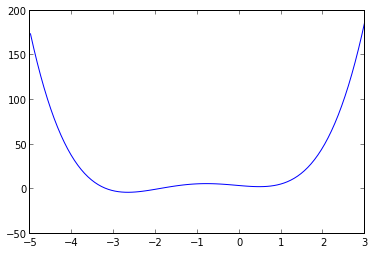We can use the fmin_bfgs function to find the minima of a function:

Optimization terminated successfully.
Current function value: -3.506641
Iterations: 6
Function evaluations: 30

array([-2.67298167])

Optimization terminated successfully.
Current function value: 2.804988
Iterations: 3
Function evaluations: 15

array([ 0.46961745])


We can also use the brent or fminbound functions. They have a bit different syntax and use different algorithms.

0.46961743402759754

-2.6729822917513886


### Finding a solution to a function

To find the root for a function of the form $f(x) = 0$ we can use the fsolve function. It requires an initial guess: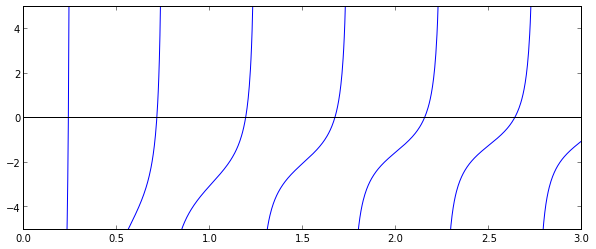array([ 0.23743014])

array([ 0.71286972])

array([ 1.18990285])


## Interpolation

Interpolation is simple and convenient in scipy: The interp1d function, when given arrays describing X and Y data, returns and object that behaves like a function that can be called for an arbitrary value of x (in the range covered by X), and it returns the corresponding interpolated y value: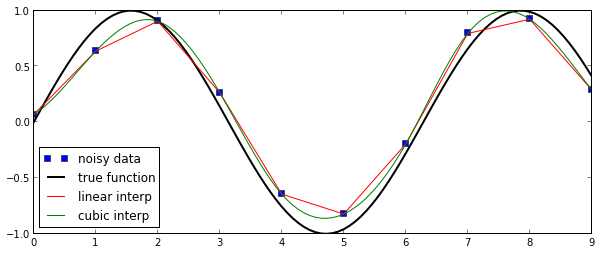## Statistics

The scipy.stats module contains a large number of statistical distributions, statistical functions and tests. For a complete documentation of its features, see http://docs.scipy.org/doc/scipy/reference/stats.html.

There is also a very powerful python package for statistical modelling called statsmodels. See http://statsmodels.sourceforge.net for more details.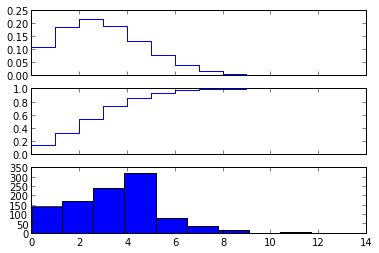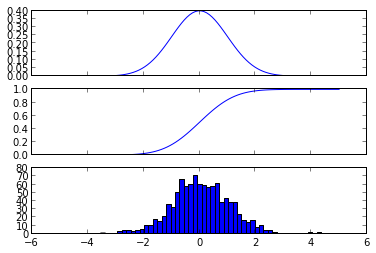Statistics:

(3.5, 1.8708286933869707, 3.5)

(0.0, 1.0, 1.0)


### Statistical tests

Test if two sets of (independent) random data comes from the same distribution:

t-statistic = -0.244622880865
p-value = 0.806773564698


Since the p value is very large we cannot reject the hypothesis that the two sets of random data have different means.

To test if the mean of a single sample of data has mean 0.1 (the true mean is 0.0):

(-4.4661322772225356, 8.8726783620609218e-06)


Low p-value means that we can reject the hypothesis that the mean of Y is 0.1.

0.0

(0.51679431628006112, 0.60541413382728715)


• http://www.scipy.org - The official web page for the SciPy project.
• http://docs.scipy.org/doc/scipy/reference/tutorial/index.html - A tutorial on how to get started using SciPy.
• https://github.com/scipy/scipy/ - The SciPy source code.

## Versions

SoftwareVersion
Python3.3.2+ (default, Feb 28 2014, 00:52:16) [GCC 4.8.1]
IPython2.2.0
OSposix [linux]
numpy1.8.2
scipy0.14.0
Tue Aug 26 22:45:19 2014 JST

Tags:

Categories:

Updated: